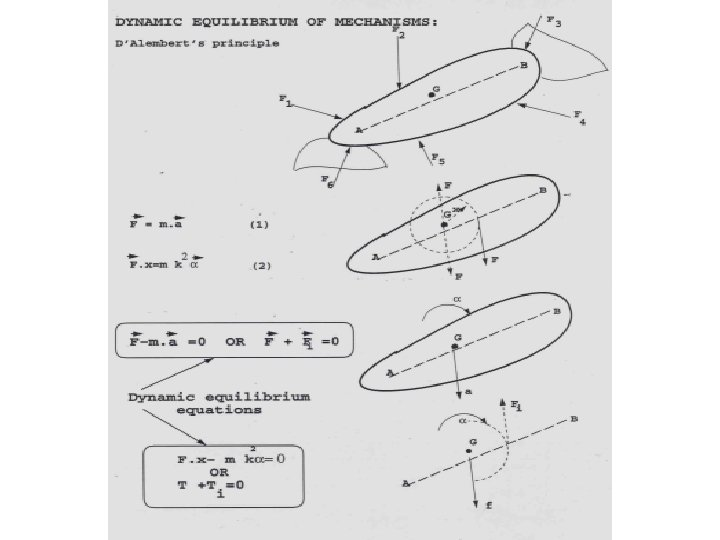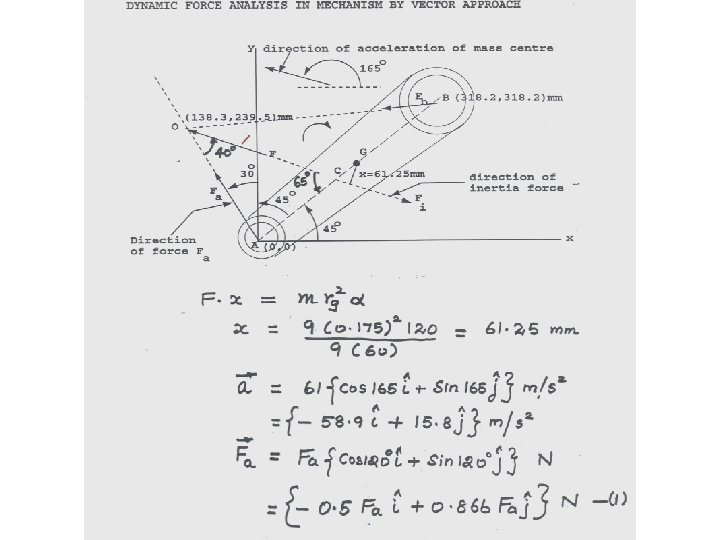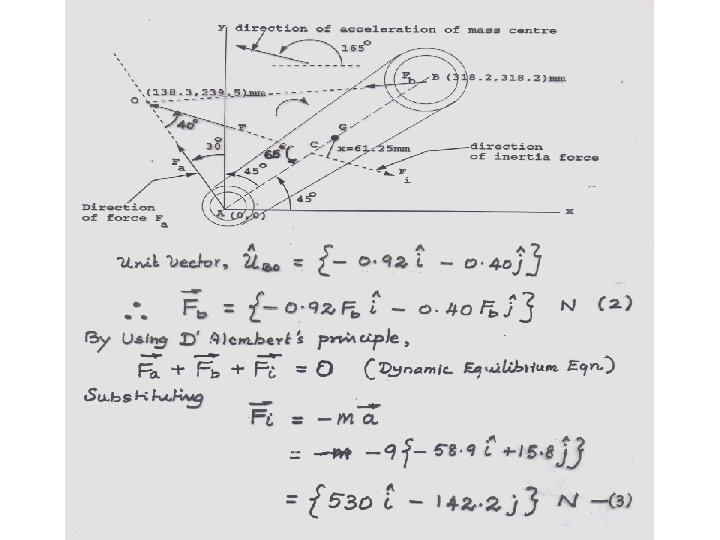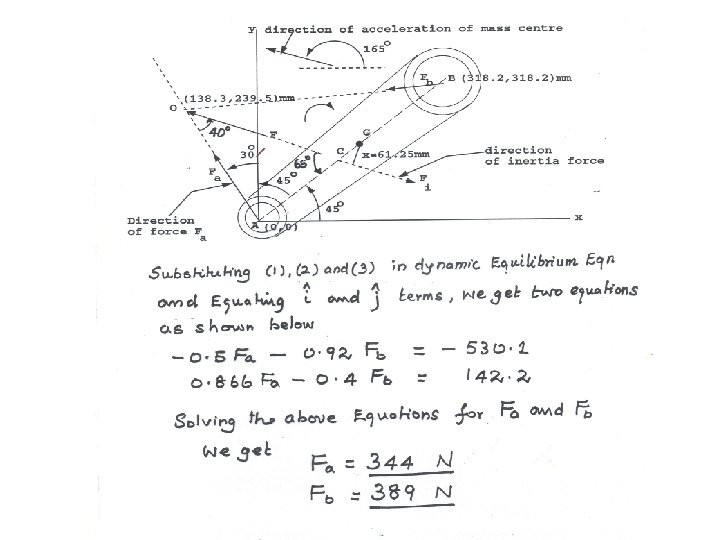# DYNAMICS OF MACHINES To know the purpose for

• Slides: 18DYNAMICS OF MACHINES • To know the purpose for which the m/c is needed. • To develop concept design. • To analyze the motion involved - Kinematics • To calculate & analyze the forces and torques required for producing the motion - Kinetics • To give physical shape to the components. - Machine DesignSTATIC FORCE ANALYSIS IN MACHINES • To evaluate the forces acting on the machine members at a particular configuration. • Each configuration is considered as system of rigid bodies in static equilibrium under the action of external forces. • Helps in finding load carried by each part of the machine during cycle of its operationTYPES OF FORCES • Applied forces : Gas, hydraulic & pneumatic forces etc. . Electrical, magnetic & gravity forces • Constraint forces : Reaction forces. • Friction forces. • Inertia forces. Due to mass of accelerating parts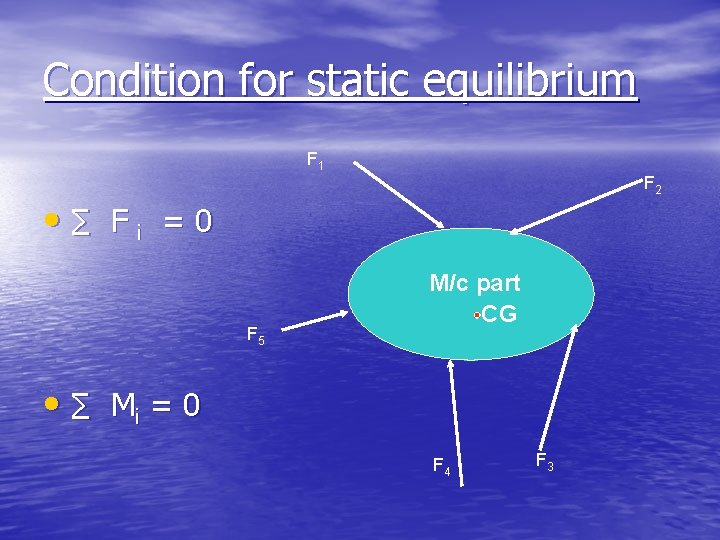Condition for static equilibrium F 1 F 2 • ∑ Fi =0 F 5 M/c part CG • ∑ Mi = 0 F 4 F 3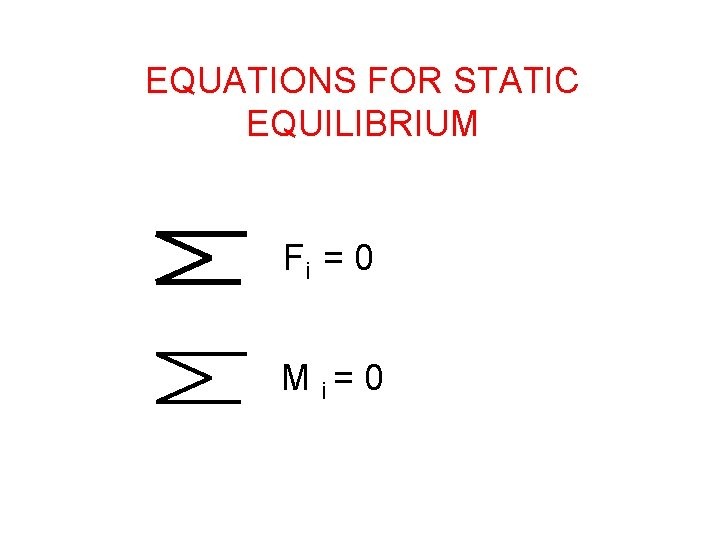EQUATIONS FOR STATIC EQUILIBRIUM Fi = 0 M i= 0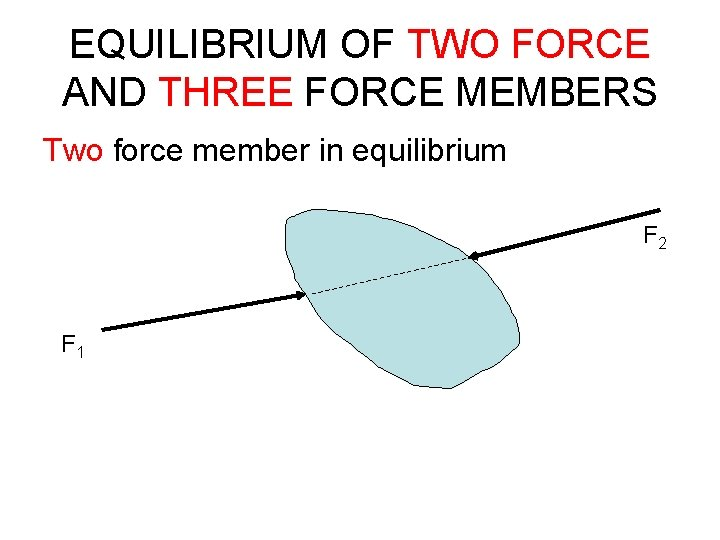EQUILIBRIUM OF TWO FORCE AND THREE FORCE MEMBERS Two force member in equilibrium F 2 F 1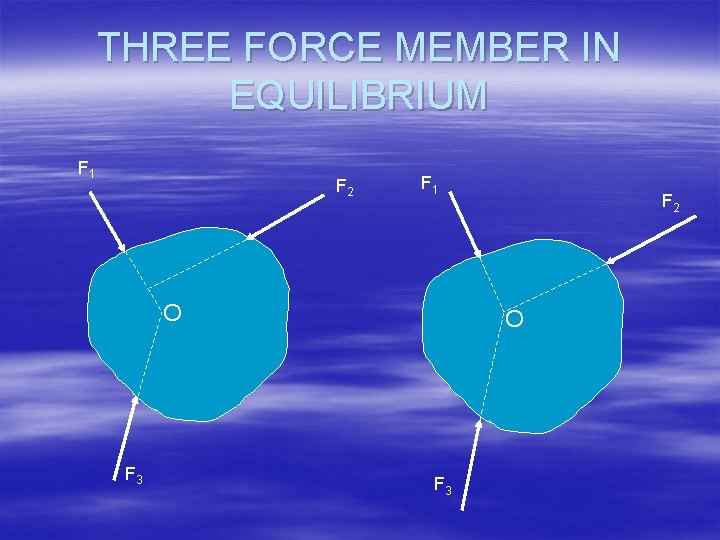THREE FORCE MEMBER IN EQUILIBRIUM F 1 F 2 F 1 O F 3 F 2 O F 3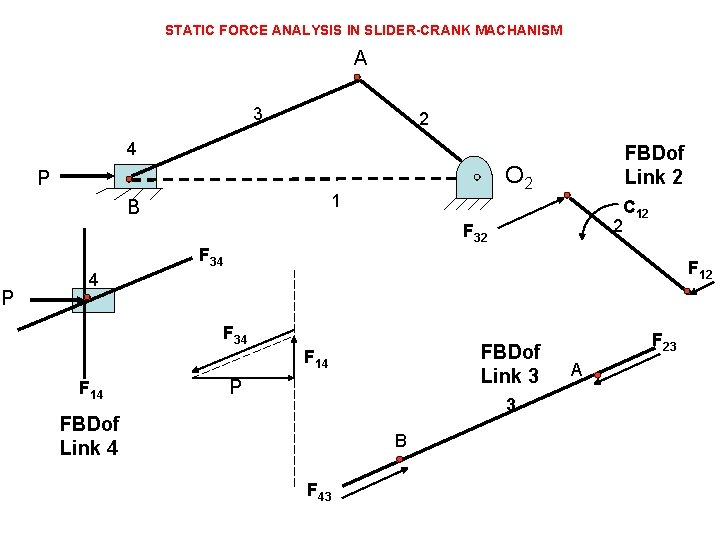STATIC FORCE ANALYSIS IN SLIDER-CRANK MACHANISM A 3 2 4 O 2 P 1 B FBDof Link 2 C 12 2 F 34 P F 12 4 F 34 FBDof Link 3 F 14 P 3 FBDof Link 4 B F 43 F 23 A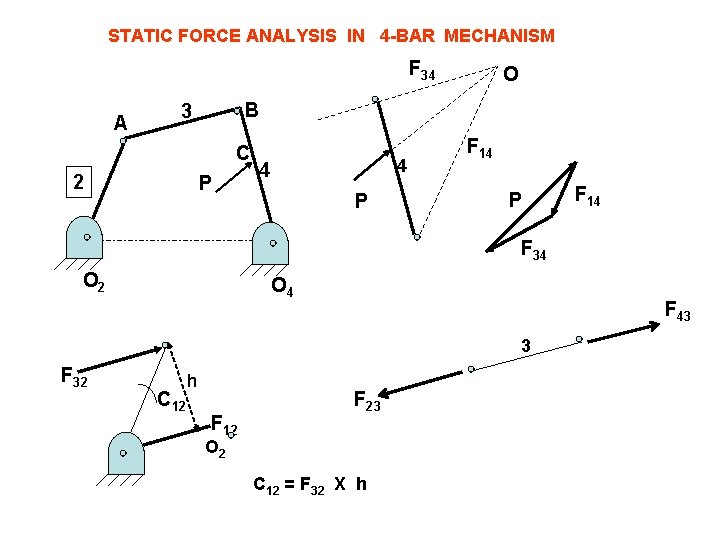STATIC FORCE ANALYSIS IN 4 -BAR MECHANISM F 34 A B 3 C 2 O P 4 4 P F 14 F 34 O 2 O 4 F 43 3 F 32 C 12 h F 12 F 23 O 2 C 12 = F 32 X hP O 2 6 5 C B 3 P A 2 6 C 5 O 2 4 F 16 1 P O 4 F 56 F 16 F 56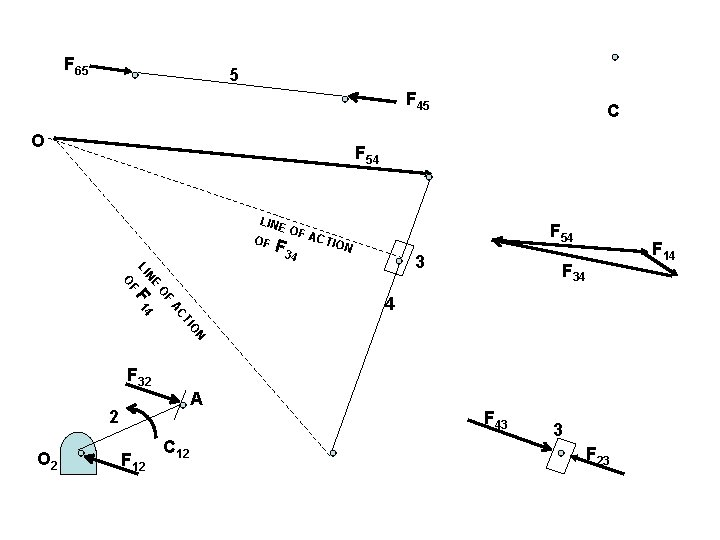F 65 5 F 45 O C F 54 LINE OF OF A F 3 4 F 54 CTIO N 4 AC F 14 OF OF F 34 NE LI 3 ON TI F 32 A 2 O 2 F 12 C 12 F 43 3 F 23 F 14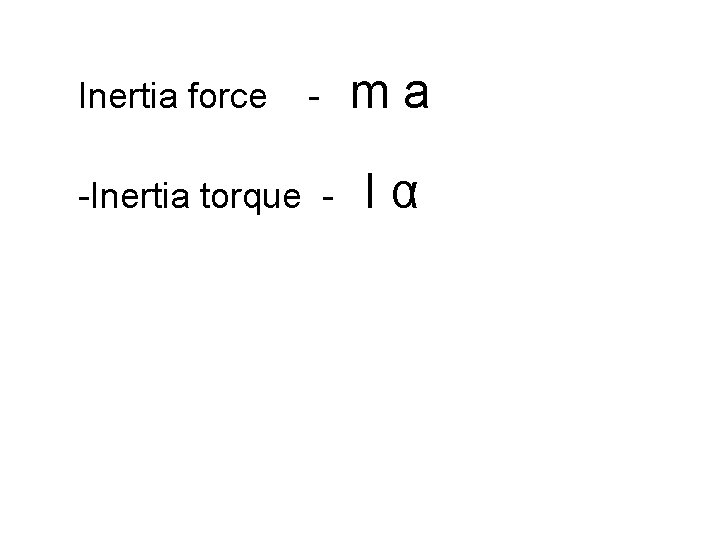Inertia force - -Inertia torque - ma Iα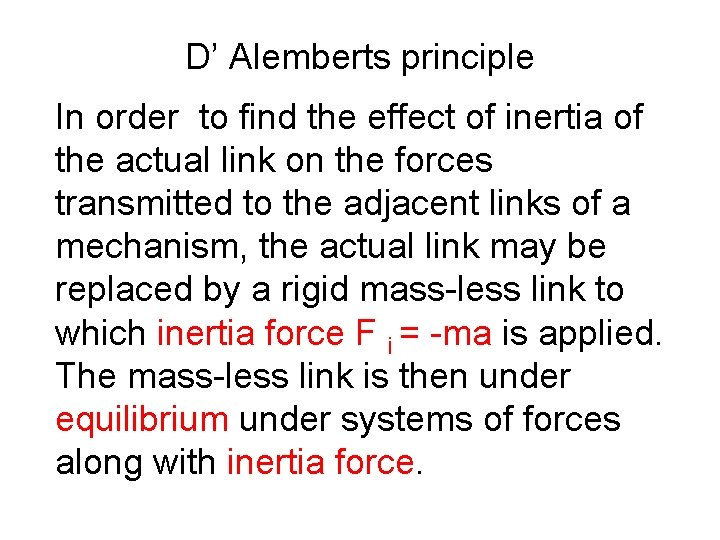D’ Alemberts principle In order to find the effect of inertia of the actual link on the forces transmitted to the adjacent links of a mechanism, the actual link may be replaced by a rigid mass-less link to which inertia force F i = -ma is applied. The mass-less link is then under equilibrium under systems of forces along with inertia force.